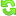Hello Learner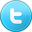Contributor
Stats
 Views : 19704 Comments : 0 Rating : 0
Actions

# Program to print rectangle with stars using C#.NET

By Ashok Nalam on 23 Dec 2013 | Category: C# | Tagged: number .NET
The Program to prints rectangle with stars using C#.NET.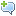Add Comment   |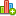Rate this resource

#### Introduction:

One of friend requested to site "I want a program that printing a rectangle with stars using two for loops and one if else". The snippet shows how to prints rectangle with stars using C#.NET.

Rectangle:
A rectangle is a four-sided flat shape where every angle is a right angle (90°) and opposite sides are parallel to each other. If four sides are equal then it's called square.

#### Program:

```using System;
namespace DotNetMirror
{
class PrintRectangleStars
{
static void Main()
{
Console.WriteLine("Program to Print Rectangle *'s");
int width = 0, height = 0;

Console.Write("Enter Height :");

Console.Write("Enter Width :");

for (int i = 1; i <= height; i++)
{
for (int j = 1; j <= width; j++)
{
if ((i == 1 || i == height) || (j == 1 || j == width))
Console.Write("*"); //prints at border place
else
Console.Write("\$"); //prints inside other than border
}
Console.WriteLine();
}
}
}
}
```

#### Output: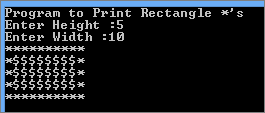Add CommentRate this resource
 Name Email WebSite